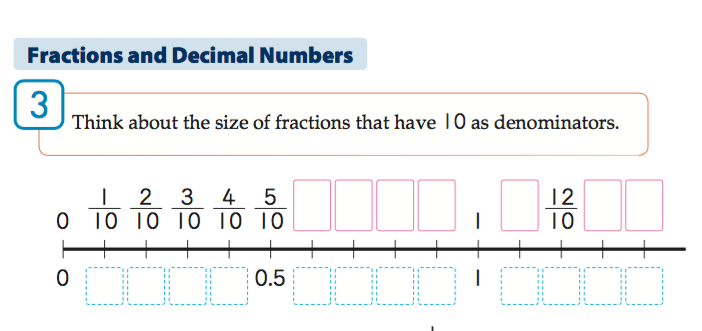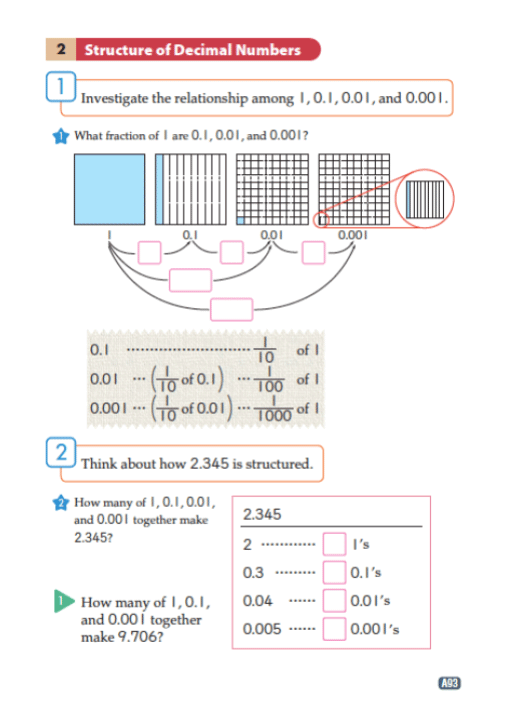# Decimals

## Overview

In this resource, we discuss the teaching and learning of decimals. We consider important similarities and differences between decimals and fractions, qualities of our base-ten number system to emphasize when teaching decimals, and considerations for selecting models to represent decimals.

## Decimals and Fractions

Decimal numbers and fractions are two representations useful to express numbers and quantities that involve amount that is less than the unit of 1. We often need to express such quantities in the context of measurement, and that is the reason most Japanese elementary mathematics textbooks introduce decimal numbers and fractions in measurement contexts.Reproduced with permission from Tokyo Shoseki publishing company, Japan.

Mathematics International Grade 3, p. B13, p. B43

One way to deal with such a situation is to introduce a smaller unit. However, no matter how small a new unit is, it may still not be small enough, and you may have to keep coming up with a new unit. Moreover, expressing a measured quantity using multiple units can be a bit cumbersome. It is a lot simpler if we can say 2.388 meters instead of 2 meters, 38 centimeters, and 8 millimeters.

Although decimal numbers and fractions are both used to express fractional numbers and quantities, each has its own merits and challenges. For example, because fractions are based on units created by more flexibly partitioning 1, it can more concisely represent quantities like 1/3. However, we need different ways to calculate with fractions. For example, we must use a common denominator to add or subtract fractions. With decimals, on the other hand, we can extend the algorithms we learned with whole numbers because they are based on place values.

Although both fractions and decimal numbers are based on fractional units, there is a major difference between them as systems of written numbers. With fractions, the numerators count the number of fractional units (of the size indicated by the denominator). The counting can go on beyond the denominator in the case of improper fractions. On the other hand, decimal numbers must follow the grouping by 10 principle, and once you reach 10 of a decimal unit, they must be exchanged. As we will discuss later on, writing “10” in the tenths place will not work.Reproduced with permission from Tokyo Shoseki publishing company, Japan.

Mathematics International Grade 3, p. B50

## Decimals and the Base-10 Number System

While the units of fractions are created by equally partitioning 1 into however many parts, new units of decimal numbers are always created by partitioning the smallest unit (at that point) into ten equal parts. (As an example, the first decimal unit is tenths, which is created by partitioning 1 into ten equal parts). By maintaining the 1 to 10 relationship, we can extend the base-10 number system to the right of the ones place. Because decimal numbers are an extension of the base-10 number system, students’ learning of decimal numbers should reinforce their understanding of the principles of the base-10 number system. The principles are as follows:

1. It is a positional system – each place represents a value (or a unit), and a numeral represents how many of the unit there are. That is, the same numeral written in different places represent different numbers.
2. It is based on grouping by 10 – when you have 10 of the same unit, you exchange it with 1 of the next larger unit. Therefore, the place values are powers of 10.
3. We must write one and only one numeral in each place – we sometimes have to use 0 as a placeholder. The leading 0s in whole numbers and the trailing 0s in decimal numbers are not considered placeholders.

Students in Grades K-3 should have an understanding of these principles from learning about increasingly larger numbers (starting at single digits in Kindergarten and progressing to numbers with four digits in Grade 3) and from learning how to calculate with these numbers. For example, regrouping while adding multi-digit numbers is needed because of the second principle above; we cannot add “12” in the ones place when adding 35+27.

Just as 10 is made up of ten 1s and 100 is made up of ten 10s, and so on, it is also possible to think of 1 being made up of ten of a smaller unit. Because students in the United States are typically introduced to fractions before decimal numbers, we can use the fraction language to talk about this unit (or the place value) being one-tenth–that is, one of ten equally divided parts of 1.

With whole numbers, we always use the right-most place of a written number as the one’s place. If we are to extend the number system to include the tenth’s place to the right of the one’s place, we need to somehow indicate where the one’s place is. That’s the reason we introduce the decimal point to indicate that the place to the left of the decimal point is the one’s place and to the right is the tenth’s place.

### Decimal Places Are Units

Once the place value and the decimal point notation is established, students should understand, for example, 0.3 means there are 3 0.1-units, or 3 tenths, just as 3 in the tens place indicate 3 tens. By understanding this principle, students can extend their understanding of addition and subtraction to perform calculations such as 0.3 + 0.4 or 0.8 – 0.5 by thinking about these numbers in terms of units of 0.1. This is parallel to what they have done when they used 10’s and 100’s as a unit to calculate 300 + 400 or 80 – 50, or even with fractions like 3/5 + 4/5 or 8/3 – 4/3. By realizing that they can add and subtract decimal numbers, they can understand that decimal numbers are indeed numbers just like whole numbers (and fractions, in the US context). To further develop this understanding of decimals as numbers, students should experience placing decimal numbers on a number line.

### What Should Students Know About Place Value?

In the CCSS, students are expected to understand decimal numbers to the second decimal place, or the hundredths place. Thus, students need to understand that just as 1 is made up of 10 0.1-units, 0.1 is made up of 10 of a smaller unit called 0.01, or a hundredth. In other words, one of 10 equal parts of 1/10 is 1/100, and the place to the right of the tenths place is the hundredths place. One important yet implicit idea we want students to understand when we expand the decimal place from the tenths to the hundredths place is that this way of expanding the decimal places can go on to create additional decimal units. Unfortunately, that idea seems to remain implicit in the CCSS.Reproduced with permission from Tokyo Shoseki publishing company, Japan.

Mathematics International Grade 4, p. A89

In the Japanese curriculum, students experience the extension of the decimal unit an additional time. They are introduced to decimals in Grade 3 by learning that it is possible to extend the base-10 number system to the right of the ones place, resulting in tenths. Then, in Grade 4, they extend the system further to the right by learning about the second and third decimal places, that is the hundredths and thousandths places. By extending the decimal places further to the right in Grade 4, students may conclude that this system can be extended further to the fourth, fifth, and so on, decimal places even though the textbooks do not explicitly discuss decimal numbers that go beyond 3 decimal places.Reproduced with permission from Tokyo Shoseki publishing company, Japan.

Mathematics International Grade 4, p. A93

## Modeling Decimals

In the United States, it is common to see textbooks and teachers use money as a model for decimal numbers. Although the monetary notation system appears similar to the base-10 number system, very few people actually think of \$3.45 as “three point four five dollars,” or “three and forty-five hundredths dollars.” Rather they think of it as “three dollars and 45 cents.” Thus, the dot in the monetary system simply indicates what follows is in the unit of cents. In essence, in \$3.45, two whole numbers, 3 and 45, are joined together by a dot. We rarely think of “4” in \$3.45 by itself. Moreover, monetary amounts are always written using both “decimal” places even if the hundredth place is a zero (e.g. \$2.30 would never be represented as \$2.3). The base-10 number system, on the other hand, says we omit the trailing 0. (This was our third principle above).

So then, if not using money, how should decimals be introduced? Is there an alternative model that should be used instead? While careful consideration of models is important later on, I would argue that the best way to introduce decimals is by having students encounter situations where decimal numbers (or fractions) are needed. This allows them to make meaning of decimal numbers rather than base their understanding on a model of decimal numbers that is for someone who already understands them.

As noted at the beginning, decimal numbers are useful to express quantities that include amounts less than 1. Thus, contexts used to introduce decimal numbers should involve continuous quantities, not discrete quantities based on counting. Expressing 1.5 centimeters makes sense but 1.5 cars does not, for example. Continuous quantities can commonly be found in measurement contexts, so this may be a good way to introduce decimals.

Certain measurement contexts lend themselves better as an introduction to decimal numbers than others. The context should be in a measurement attribute that students are familiar with and that lends itself to easily comparing the fractional quantity to the unit. By Grade 4, students have learned measurable attributes of length, weight (mass), elapsed time, area, and liquid volume. In Grade 4, they also learn angle and volume, but those attributes may be too new for students to use to explore a new mathematical idea. Comparing fractional amount to the unit can be difficult with elapsed time and weight (mass). Thus, we are left with length, liquid volume or area. In order to discuss decimal numbers, we want students to be able to easily handle 0.1 unit. Therefore, we want the unit to be reasonably large so that the 0.1 unit is still a manageable size. Considering all these factors, linear measurement with meter as the unit or liquid volume with liter as a unit may be the most convenient contexts to introduce decimal numbers.

Once they understand what decimal numbers are, then base-10 blocks, number disks (with units of 0.1), abacus, number line, etc. may be perfectly appropriate models of decimal numbers. What specific idea about decimal numbers you want your students to learn should be considered when deciding on which model to use. One thing teachers might want to keep in mind is that initial models of decimal numbers should be proportional; that is, the model of 1 should be 10 times as big as the model of 0.1. From this perspective, base-10 blocks are useful concrete materials. In fact, if you are to use a liquid volume context to introduce decimal numbers, a 1000-block is the actual size of 1 L (1000 cm3). So, using a 1000-block representing 1 (L) and a flat representing 0.1 (L) might also provide students a concrete referent for how big 1 L is.

## Discussion Questions

After reading this resource, it may be helpful to discuss the following questions as a team.

1. What ideas about the teaching and learning of decimals strike you as important in your setting?
2. How does your curriculum handle the topic of decimals? What about it do you like and/or notice that has the opportunity for improvement?
3. What have your students learned about fractions and the base-ten number system that might be relevant to their understanding of decimals?